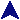Text to HTML TranslatorCode Examples```#
# Translate a simple langauge into HTML.
#   Blank lines become <p>
#   _stuff_ becomes <u>stuff</u>
#   @stuff@ becomes <tt>stuff</tt>
#   {stuff} becomes <i>stuff</i>
#   [stuff] becomes <b>stuff</b>
#   [[stuff]] becomes <h1> header.
#   [:stuff:] becomes <h1> header and centered.
#   A line of 3 or more --- becomes an <hr>.
#   A >>> at the start of a line becomes &nbsp;&nbsp;&nbsp;&nbsp;&nbsp;
#   Other stuff is retained, with & < > " being translated into
#   approprite stuff.  The \ escapes substitutions.
#   Generally, \ is removed.  Use \\ to add a single one.
#

use strict;

# Set of plain replacements.  Order is important.
my @plain = ( '\&', '&amp;',
'^\s*\>\>\>\s*', '&nbsp;&nbsp;&nbsp;&nbsp;&nbsp;',
'\<', '&lt;', '\>', '&gt;', '\"', '&quot;',
'\[\[', '<h1>', '\]\]', '</h1>',
'\[\:', '<center><h1>', '\:\]', '</h1></center>',
'\{', '<i>', '\}', '</i>',
'\[', '<b>', '\]', '</b>',
'^\s*\$', '<p>',
'^\-\-\-+\$', '<hr>' );

# Set of replacements which toggle.  Replacement will be the open
# or closing version of the tag.
my @toggle = ( '\_', 'u', '\@', 'tt' );
my %toggle_status = ( '\_' => '', '\@' => '' );

# Prefix.
print '<html><body bgcolor="white">', "\n";

# Read the input.
while(defined(my \$line = <STDIN>)) {
chomp \$line;

# Do the simple replacements.
my @rs = @plain;
while(my \$r = shift @rs) {
my \$rt = shift @rs;

\$line =~ s/(^|[^\\])\$r/\$1\$rt/g;
}

# Work through the toggles.
@rs = @toggle;
while(my \$r = shift @rs) {
my \$rt = shift @rs;

# Accumulate the new line here.
my \$newline = '';

# Take each left-most match for \$r.  The *? construct
# is a * that matches as few as possible.
while(\$line =~ /^(.*?(^|[^\\]))\$r(.*)\$/) {
# Move the left part to newline and add the
# replacement, then continue with the right part.
\$newline .= "\$1<\$toggle_status{\$r}\$rt>";
\$line = \$3;

# Toggle the status of the symbol.
\$toggle_status{\$r} = \$toggle_status{\$r} ? '' : '/';
}
\$line = \$newline . \$line;
}

# Reduce escapes.
\$line =~ s/\\(.)/\$1/g;

print "\$line\n";
}

print "</body></html>\n";
```